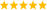LabVIEW

cancel
Showing results for
Did you mean:

Solved!
Go to solution
Highlighted

Matrix - sum of elements under/above main diagonal

I tried to add the elements from the main diagonal with this code ( replace < with = ) and it works...If I use < or > the code won't work and I saw that it depends which element from the index ( inside the second for ) I add .Can someone explain me why it depends which element I add ? Why are there 2 elements in the first place and not one element with the i,j coordinates ?

I want an answer for this solution,I'm not interested in other much simpler solutions.

How can I make THIS code easier ?

Message 1 of 6
(1,323 Views)

Re: Matrix - sum of elements under/above main diagonal

• Before attaching code, fill the matrix control with typical values and make them the default. An empty matrix means more work for us and we have to make assumptions, e.g. Is the matrix square?
• Always save a VI under a reasonable name related to the problem. Over time, my downloads folder contains so many "untitled 1.vi" files that I am up to "utitled 1(10).vi. now. This often breaks things, for example if a subVI is named this way, the caller most likely calls some stale old VI.Regarding your problem, try some debugging steps, for example place some probes or use execution highlighting. Slow down the loops and add extra indicators, etc.

Since you are autoindexing on the outer loop, you only have a 1D array inside the inner loop and you are indexing out two neighbors. If you would disable indexing on the outer loop input, you'll get a 2D array and can get a single element based on the two indices. Do some tutorials!LabVIEW ChampionDo more with less code and in less timeMessage 2 of 6
(1,310 Views)

Re: Matrix - sum of elements under/above main diagonal

@dafuq313 wrote:

I want an answer for this solution,I'm not interested in other much simpler solutions.

@dafuq313 wrote:

How can I make THIS code easier ?

You are contradicting yourself. Easier is always simpler. The explanation is at the end of my previous post. You need to understand autoindexing and arrays. Once you understand it, the code boils down to this.LabVIEW ChampionDo more with less code and in less timeMessage 3 of 6
(1,292 Views)
Solution
Accepted by topic author dafuq313
11-11-2016 12:43 PM

Re: Matrix - sum of elements under/above main diagonal

@dafuq313 wrote:

I want an answer for this solution,I'm not interested in other much simpler solutions.

Here's how to program your solution correctly. (of course since the matrix is always square, we only need to get one of the dimensions...)LabVIEW ChampionDo more with less code and in less timeMessage 4 of 6
(1,273 Views)

Re: Matrix - sum of elements under/above main diagonal

Thank you for answers,I was trying to say that there are easier solutions ( withouth 2 fors ) and I didn't want one of those.

I couldn't find proper tutorials for labview since is not that much used...

Thank you very muchMessage 5 of 6
(1,219 Views)

Re: Matrix - sum of elements under/above main diagonal

Even my simple solution has two FOR loops.LabVIEW ChampionDo more with less code and in less timeMessage 6 of 6
(1,200 Views)